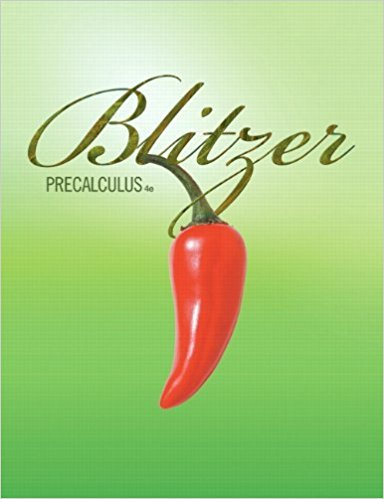×
×

# Solutions for Chapter 1.10: Modeling with Functions## Full solutions for Precalculus | 4th Edition

ISBN: 9780321559845Solutions for Chapter 1.10: Modeling with Functions

Solutions for Chapter 1.10
4 5 0 347 Reviews
19
2
##### ISBN: 9780321559845

Precalculus was written by and is associated to the ISBN: 9780321559845. This textbook survival guide was created for the textbook: Precalculus, edition: 4. Chapter 1.10: Modeling with Functions includes 73 full step-by-step solutions. Since 73 problems in chapter 1.10: Modeling with Functions have been answered, more than 67311 students have viewed full step-by-step solutions from this chapter. This expansive textbook survival guide covers the following chapters and their solutions.

Key Calculus Terms and definitions covered in this textbook
• Arrow

The notation PQ denoting the directed line segment with initial point P and terminal point Q.

• artesian coordinate system

An association between the points in a plane and ordered pairs of real numbers; or an association between the points in three-dimensional space and ordered triples of real numbers

• Boundary

The set of points on the “edge” of a region

• Characteristic polynomial of a square matrix A

det(xIn - A), where A is an n x n matrix

• Chord of a conic

A line segment with endpoints on the conic

• Compound interest

Interest that becomes part of the investment

• Constant function (on an interval)

ƒ(x 1) = ƒ(x 2) x for any x1 and x2 (in the interval)

• Control

The principle of experimental design that makes it possible to rule out other factors when making inferences about a particular explanatory variable

• Dependent event

An event whose probability depends on another event already occurring

• End behavior

The behavior of a graph of a function as.

• Implied domain

The domain of a function’s algebraic expression.

• Line of symmetry

A line over which a graph is the mirror image of itself

• Linear programming problem

A method of solving certain problems involving maximizing or minimizing a function of two variables (called an objective function) subject to restrictions (called constraints)

• Median (of a data set)

The middle number (or the mean of the two middle numbers) if the data are listed in order.

• n-set

A set of n objects.

• Parametric equations for a line in space

The line through P0(x 0, y0, z 0) in the direction of the nonzero vector v = <a, b, c> has parametric equations x = x 0 + at, y = y 0 + bt, z = z0 + ct.

• Power function

A function of the form ƒ(x) = k . x a, where k and a are nonzero constants. k is the constant of variation and a is the power.

• Product rule of logarithms

ogb 1RS2 = logb R + logb S, R > 0, S > 0,

• Quartic function

A degree 4 polynomial function.

• Range of a function

The set of all output values corresponding to elements in the domain.

×